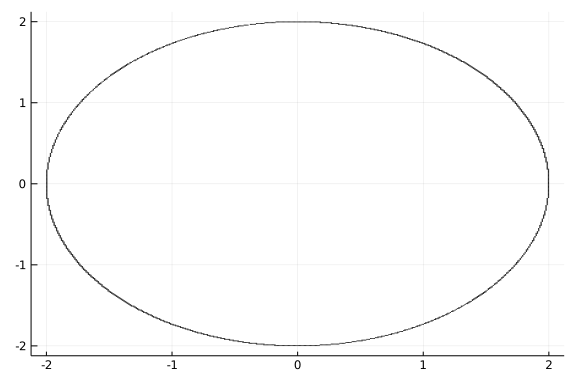# Implicit Differentiation

## Graphs of equations

An equation in $y$ and $x$ is an algebraic expression involving an equality with two (or more) variables. An example might be $x^2 + y^2 = 1$.

The solutions to an equation in the variables $x$ and $y$ are all points $(x,y)$ which satisfy the equation.

The graph of an equation is just the set of solutions to the equation represented in the Cartesian plane.

With this definition, the graph of a function $f(x)$ is just the graph of the equation $y = f(x)$.

In general, graphing an equation is more complicated than graphing a function. For a function, we know for a given value of $x$ what the corresponding value of $f(x)$ is through evaluation of the function. For equations, we may have 0, 1 or more $y$ values for a given $x$ and even more problematic is we may have no rule to find these values.

To plot such an equation in Julia, we can use the ImplicitEquations package, which is loaded when CalculusWithJulia is:

using CalculusWithJulia
using Plots
gr()   # better graphics than plotly() here

Plots.GRBackend()


To plot the circle of radius $2$, we would first define a function of two variables:

f(x,y) = x^2 + y^2

f (generic function with 1 method)


Then we use one of the logical operations - Lt, Le, Eq, Ge, or Gt - to construct a predicate to plot. This one describes $x^2 + y^2 = 2^2$:

r = Eq(f, 2^2)

ImplicitEquations.Pred(Main.##WeaveSandBox#652.f, ==, 4)


These "predicate" objects can be passed to plot for visualization:

plot(r)Of course, more complicated equations are possible and the steps are similar - only the function definition is more involved. For example, the Devils curve has the form

$~ y^4 - x^4 + ay^2 + bx^2 = 0 ~$

Here we draw the curve for a particular choice of $a$ and $b$. For illustration purposes, a narrower viewing window than the default of $[-5,5] \times [-5,5]$ is specified below using xlims and ylims:

a,b = -1,2
f(x,y) =  y^4 - x^4 + a*y^2 + b*x^2
plot(Eq(f, 0), xlims=(-3,3), ylims=(-3,3))